# Teachers' Mathematics: A Collection of Content Deserving to Be a Field

### A Mathematics Teacher Needs to Know Mathematics

Let's begin with a truism. To teach well, a teacher of mathematics should know a great deal of mathematics. The higher the level taught, the more the teacher needs to know. For a teacher of middle school or high school mathematics, this means knowing a good deal of algebra, geometry, analysis, statistics, number theory, computer science, and mathematical modeling. This mathematics constitutes the traditional background of a teacher who is considered to be well-prepared mathematically.

In the latest National Assessment data at the 8th grade level, students of teachers with a mathematics major or minor per- formed higher than students of teachers who had not majored or minored in mathematics. There are important reasons be- yond student performance why teachers need to know more advanced mathematics: one of the main reasons we teach mathematics is to prepare students who need to have an advanced knowledge of mathematics for their careers. Teachers need to know the various ways in which the mathematics they teach is applied later and to distinguish those ideas that are fundamental from those that are enrichment. Deductive proof and statistical inference have some similarities, but also they have some fundamental differences. The discrete mathematics used in computer science is different from the mathematics covered in most engineering courses. And teachers need to know the different ways in which people who use mathematics approach problems.

Even though taking more and more mathematics would not seem to have any down side, it can create a problem. Often the more mathematics courses a teacher takes, the wider the gap between the mathematics the teacher studies and the mathematics the teacher teaches. The result of the mismatch is that teachers are often no better prepared in the content they will teach than when they were students taking that con- tent. A beginning teacher may know little more about logarithms or factoring trinomials or congruent triangles or volumes of cones than is found in a good high school text. Furthermore, there is a good deal of mathematics that teachers need to know that, for reasons I will explain below, they never encounter. It may be because of this mismatch that some studies have found that the number of mathematics courses taken by a teacher does not influence the performance of the students of that teacher.

The question is how to describe this mathematics, which I call teachers' mathematics because it is particularly important for teachers to know. Some colleges identify it by the corresponding course the teacher teaches, such as teachers' algebra, or teachers' geometry, or calculus for teachers. These names have the advantage of being attractive to anyone who is teaching those courses in schools. But they have several disadvantages. They hint that the teacher probably does not know that mathematics well. Because there are other courses in algebra and geometry at most colleges, identifying a course as being "for teachers" suggests that the teachers' version is weaker. Finally, simply saying that a course is for teachers does not indicate why it is for teachers nor tell you what in the course makes it appropriate for teachers.

Obviously, whatever name is given to the course is not important compared to what is in it. I would like to argue that a teacher needs at least three kinds of mathematics not found in typical college mathematics courses. The remainder of these remarks elaborates on what these are.

### Mathematical Generalizations and Extensions

The first kind of mathematical knowledge needed by teachers consists of extensions and generalizations of the content of school mathematics. For instance, as we all know, the area of a triangle can be found by the formula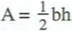where b is the base and h is the height of the triangle using that base. This is the most commonly taught formula for the area of a triangle even though the height of a triangle is not one of the sides or angles of a triangle, not one of the parts of a triangle used to prove congruence.

Almost all high school geometry textbooks, including UCSMP Geometry, mention a second formula, Hero's formula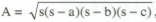which has the advantage of giving a triangle's area in terms of its three sides. Hero's formula is rather difficult to prove. For this reason, a proof of Hero's formula is seldom found in today's books even when the formula is presented. But teachers should see a proof.

Quite a bit less known, because trigonometry is studied after geometry, is that another area formula for a triangle is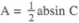. We have this formula in Functions, Statistics, and Trigonometry. This gives the area of a triangle in terms of two sides and an included angle. From this formula, it is easy to prove that the maximum area of a triangle with sides of known lengths a and b is when they are legs of a right triangle. We can think of Hero’s formula and the formulaas SSS and SAS are formulas. They motivate us to look for an area formula for a triangle given AAS or ASA. One such formula is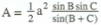. I leave the proof for you.

What benefit is there in knowing these formulas? This knowledge changes our view frombh as the formula for the area of a triangle or the most important formula to one of the many formulas for the area. Knowing many formulas shows how the different combinations of elements that determine a triangle can be employed to determine its area. It gives us another reason to learn some trigonometry. This knowledge piques our curiosity for still more formulas. My own favorite triangle area formula is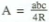, where R is the radius of the circle containing the three vertices of the triangle.

These triangle area formulas extend typical content. Now for an example of generalizing content. UCSMP Precalculus and Discrete Mathematics contains a few theorems that to my knowledge are not found in other books. Although we wrote them for students, they are just as valuable for teachers. They are meant to bring together a number of properties used in solving equations and inequalities that students have learned separately. They are also used to explain these properties. For all real numbers a, b, and c:

Addition Property of Equality: a = b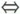a + c = b + c.
Multiplication Property of Equality: If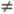, then (a = bac = bc). (Andis necessary!)
Squaring non-Property (!): If you square both sides of an equation, or take the square root of both sides of an equation, you may gain or lose solutions.
Cubing: a = ba3 = b3.
Exponential Equations: If c > 0, then (a = bca = cb).

Trigonometric Equations: If 0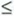a90° and 0b90°, then (sin a = sin ba = b) and (cos a = cos ba = b), but one of these is not true if 0a180° and 0b180°.

In English, we say that you can add the same number to both sides of an equation, or multiply both sides of an equation by the same non-zero number, and the resulting equation is equivalent to the given one. But if you square both sides of an equation, you may gain solutions. And cubing both sides of an equation does not affect the solutions. These results, which may seem to students to be inconsistent, suggest other questions. Does taking the log of both sides affect solutions to an equation? What about taking the sine of both sides? How can one tell, in general, whether an operation applied to both sides of an equation will change the solutions to the equation?

The answer to this question is found by considering an equation in one variable as being of the form f(x) = g(x). Applying an operation to both sides is like applying a function h both sides. This results in the equation h(f(x)) = h(g(x)).

The general theorem is:

On a particular interval, the equations f(x) = g(x) and h(f(x)) = h(g(x)) are equivalent if and only if on that interval h is a one-to-one function on the ranges of f(x) and g(x).

Examining this theorem and its special cases unifies the solving of equations and gives new insight into the process of equation-solving.

The corresponding general theorem for inequalities explains not only when inequalities are equivalent but also why the sense of the inequality changes at some times but not at others.

On a particular interval, f(x) < g(x) is equivalent to h(f(x)) < h(g(x)) if and only if on that interval h is an increasing function on the ranges off(x) and g(x). f(x) < g(x) is equivalent to h(f(x)) > h(g(x)) if and only if on that interval h is a decreasing function on the ranges of f(x) and g(x).

These are only a few of the many theorems that extend or generalize properties that students are expected to know. A whole host of natural generalizations are found in abstract algebra, and many years ago I wrote some articles about them. They are typically not encountered by teachers in their college mathematics courses because college mathematics courses tend to look ahead to what is needed for graduate school rather than look back to what would be helpful in understanding pre-college mathematics.

### Concept Analysis

A second kind of mathematics that a teacher needs to know can be described as concept analysis. Those of you teaching from any of the UCSMP texts for grades 7-12 see concept analysis in the form of the SPUR model for understanding. For instance, in Transition Mathematics we present not only the properties of each of the four fundamental operations, but also the real-world situations they model, and representations such as the coordinate plane for the size change model for multiplication. We begin UCSMP Geometry with the properties and representations for several manifestations of points and lines, derived from a concept analysis of those basic elements.

Concept analysis includes alternate definitions or conceptions of mathematical ideas and their consequences, why the concepts arose and how they have changed over time, and the range of applications of the concept. Concept analysis helps teachers to help students understand new ideas. For instance, a view of powering as repeated multiplication is insufficient to handle the zero power, non-integer rational powers, or negative powers. Without a broader view of the concept of powering and its purpose, properties are merely memorized and not assimilated by students.

Concept analyses also provide responses to questions that learners have about what they are learning. Probably every teacher in this room has been asked to explain why the repeating decimal 0.99999... = 1. Sometimes this question is asked because students have an inkling that the explanation will take you off the subject of the day. And it usually does. A common explanation uses the Multiplication Property of Equality. Start with 0.33333... =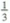. Now multiply both sides by 3. On the left side, you get 0.99999..., and on the right side you get 1. Voila!

If students appreciate this argument, they do not always show it. Instead, one student exclaims: "But 0.99999... is less than 1." So you now appeal to the Trichotomy Property of the Real Numbers and their density (without describing or naming either property). Two numbers are either equal or one is less than the other. We agree that 0.99999... is not bigger than 1. Now if 0.99999... is less than I, then there must be a number in between them. But there is no number in between them, so 0.99999... cannot be less than 1.

You see glassy stares. Undaunted, you go on, using algebra. Let's suppose that we do not know the value of 0.99999.... So we let x = 0.99999... Then 10x= 9.99999... So, subtracting the first equation from the second, 9x = 9.00000.... So x= 1. This explanation may be beyond the students, but now they begin to realize that you have many arguments, and they start to believe that 0.99999... may in fact equal 1 because you said so.

You are not finished. You decide to get directly to one meaning of infinite decimal, as a limit of finite decimals. You point out that 0.9, 0.99, 0.999, ... are all less than one, but they are getting closer and closer to the number 0.99999... You show this on the number line, and point out that 0.99999... is the limit of this sequence of finite decimals. You use the opportunity to point out that every infinite decimal is a limit of a sequence of finite decimals. And in this case the limit is 1.

If the question comes up after you have studied geometric series, then you have still another opportunity to examine this infinite repeating decimal. Now you view the infinite decimal as a sum. 0.9 + 0.09 + 0.009 +... is the sum of a geometric series with first term a = 0.9 and constant ratio r = 0.1. In general, the sum of such a series is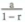so here the sum is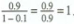This last explanation does not help students who have not seen some of the other explanations, but it does show the consistency of mathematics and helps to give closure on the idea. This is teachers' mathematics.

Our knowledge of concepts changes over time. Negative numbers are an everyday occurrence with temperatures, altitudes above and below sea level, time before and after, scientific notation, assets and debts, scores above and below par in golf, and so on. Yet even some notable mathematicians had great trouble with negative numbers. Augustus de Morgan, the logician and algebraist who lived from 1806 to 1871, centuries after negative numbers had been accepted by the mathematical community, still argued that a person only got a negative number as a solution to an equation if the equation was incorrectly set up. He illustrated this with the following problem: A father is 56 years old and his son is 29. When will the father be twice as old as the son? Let x be the number of years from now, we obtain 56+ x = 2(29 + x), and so x= -2. This is easily interpreted as a negative number of years from now, so a positive number of years ago. But de Morgan views this solution as absurd, and argues that the variable was in- correctly identified, and should have been the number of years ago, with 56 - x = 2(29 - x) as the equation. (see Helen Featherstone, '"-Pat+ Pat= 0': Intellectual Play in Elementary Mathematics", For the Learning of Mathematics 20, 2 (July 2000), pp. 14-23.)

Similarly, some contemporary mathematicians have views of algebra so narrow that they do not realize the broad range of applications of the subject. They, like many teachers, would benefit from a course that analyzes concepts for the variety of their meanings, unlike other courses which assign a meaning early and then are restricted to what can be deduced using that conception.

### Problem Analysis

A third kind of mathematics needed by teachers but rarely encountered by them, and different from concept analysis, is the analysis of problems. Teachers who are the coaches of mathematics teams and good students on these teams can be quite proficient at this kind of analysis. Problem analysis involves more than finding different ways of solving a problem. It includes looking at a problem after it has been solved and examining what has been done. Will the method of solution work for other problems? Can we extend the problem? And so forth. Let me begin with a typical problem found in algebra books:

Jane has an average of 87 after 4 tests. What score does she need on the 5th test to average 90 for all five tests?

To answer this question, the algebra student is expected to let a variable, such as x, stand for Jane's score on the 5th test and to solve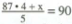. Solving the equation, we find that x = 102, so that if the last test only allows 100 points, she cannot average 90. But many students will use arithmetic, working somewhat as follows. To average 90 points on 5 tests means to have 450 points. Jane has 348 points, so that she needs 102 points. The algebra parallels this. Students who see the arithmetic solution will naturally wonder why algebra is needed, and they are right because it is not needed for that problem.

But when we inquire into the relationship between Jane's score on the 5th test and her average, we cannot avoid algebra. Let A equal Jane's average for all 5 tests.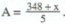If we think of x as the domain variable and A as the range variable, this is a linear function with slope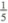. It indicates that each point Jane gets on the 5th test addsto her average. We can use this equation to determine what Jane needs to obtain any given average, not merely the average of 90. The graph of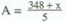shows all the possible solutions.

The problem analysis could end there, except that one im- portant aspect of this analysis is to relate this problem to simi- lar problems. The linear equation shows that this situation is a constant-increase situation. Each point Jane earns on the 5th test adds the same amount to her average.

And now we extend the problem. In April, 1998, the basketball players Michael Jordan and Shaquille O'Neal were vying for the season individual scoring title. The scoring title is won by the player with the highest average per game played. At the beginning of the day, Michael had 2313 points in 81 games, for an average of28.6 (customarily, averages in news- papers are rounded to the nearest tenth). Shaq had scored 1666 points in 59 games, for an average o f 28.2 points. With one day left in the season, no one else had a chance to win the title.

Most sports fans know that the average number of points per game is calculated by dividing the total number of points by the number of games. With this knowledge, it is easy to try out various scenarios. Just estimate how many points Jordan and Shaq score in their last game, and then calculate, on the basis of those guesses, who will win the scoring title. These calculations require only arithmetic. If there were only a couple of possibilities for the numbers of points scored, this arithmetic would give you rather quickly all of the possible outcomes. But there are many possibilities: Each player might reasonably score any number of points from 10 to 60. For this reason it is useful to consider all the scenarios at one time, and algebra is needed.

If Jordan scores j points in his last game, then he has a total of 2313 + j points in 82 games, for an average of 2313 + j points in 82 games, for an average of . Similarly if s is the number of points Shaq scores in his last game, he will then have 1666 + s points in 60 games, for an average of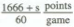. Consequently, Jordan wins the scoring title whenever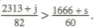On the other hand, Shaq wins the scoring title whenever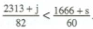I have time only to outline what can be done with this problem. First, we must ask what it means to solve inequalities like these? We cannot simply say j equals this and s equals that, because there are infinitely many solutions even if one of the variables is fixed. So we graph the boundary to the inequalities. That is the line with the equation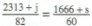. Solving for s in terms of j, s =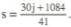(See Figure 1.)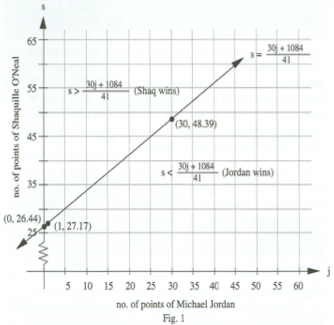Each of the points on the line has meaning. If Jordan scores 0 points, Shaq still needs to score over 26.44 points to win the title. That is, he needs 27 points or more. If Jordan scores 1 point, Shaq needs over 27.17 points - 28 points or more - to win. If Jordan scores 30 points, Shaq needs 49 points or more. We see that the lattice points above the line in the first quadrant offer all the possible ways in which Shaq wins. The lattice points below the line in the first quadrant show all the possible ways in which Jordan wins.

It happened that Jordan scored 44 points in this last game of the regular season. When j = 44, s = 58.63..., which meant that Shaq had to score 59 points in his last regular season game to win the title. That is, Shaq would need a personal record for him to win the scoring title. Shaq scored 39 points, which is terrific scoring, but was not good enough to win the scoring title.

I want to take the analysis one step further. In the Jordan-Shaq situation, two players are vying for the better average at the end of the season, rather than one student wondering what her test average would be. Suppose we let f(j) = Jordan’s average if he scores j points in the last game, and g(s) = Shaq's average if he scores s points in the last game. Above we showed that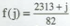, and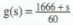. The equation of the line is when the two averages are the same; that is, it is f(j) = g(s). Since g is a linear function, it has an inverse, and so g-1 º f(j) = g-1 º g(s) = s. That is, s = g-1 º f(j). Thus the equation that we graphed, s =, is the equation of g-1 º f.

Notice the meaning of g-1 º f. The function f maps Jordan's last game points onto Jordan's season average. The function g maps the number of points Shaq gets in the last game onto his average for the season. I f the season averages are to be equal, then g·1 is mapping Jordan's average onto the number of points Shaq needs to get that average. Thus the function g·1 o f maps the number of points Jordan scores in the last game onto the number of points Shaq needs to have the same average as Jordan. In this way, function composition and function inverses both generalize and provide an explanation for a common algebra problem.

### Teachers' Mathematics

Mathematical generalizations and extensions, concept analysis, and problem analysis together constitute a substantial body of mathematics that arises from teaching situations in much the same way that statistics arises from data, financial mathematics arises from investment problems, operations research arises from optimization problems in business, and actuarial science arises out of questions of insurance. I call this body "teachers' mathematics". Teachers' mathematics includes:

1. explanations of new ideas
2. alternate definitions and their consequences
3. why concepts arose and how they have changed over time
4. the wide range of applications of the mathematical ideas being taught
5. alternate ways of approaching problems, including ways with and without calculator and computer technology
6. how problems and proofs can be extended and generalized
7. how ideas studied in school relate to ideas students may encounter in later mathematics study
8. responses to questions that learners have about what they are learning.

Teachers' mathematics includes some of what some educators have called pedagogical content knowledge, and much more because it includes a lot of mathematics that is not at all pedagogy. A course in teachers' mathematics is not a methods course, though it may suggest mathematical methods of approach to topics.

There is a huge amount of material that falls under this heading. However, this material is usually picked up by teachers only haphazardly- through occasional articles in journals, by attending conferences like UCSMP's, by reading the teachers' notes found in their textbooks, or by examining research in history and conceptual foundations of school mathematics. This mathematics is often not known to professional mathematicians. It covers both pure and applied mathematics, algorithms and proof, concepts and representations. The only way we will get this material to our teachers on a broad scale is if "teachers' mathematics" obtains its own place in the curriculum.

Teachers' mathematics is not merely a bunch of mathematical topics that might be of interest to teachers, but a coherent field of study, distinguished by its own important ideas: the phenomenology of mathematical concepts; the extended analyses of related problems; and the connections and generalizations within and among the diverse branches of mathematics. Teachers' mathematics is a branch of applied mathematics, applied because it emerges directly from problems in the classroom. Teachers' mathematics comes out of the teaching and learning of mathematics. The importance of teachers' mathematics thus goes well beyond the need of teachers to include all those who study the learning of mathematics and the mathematics curriculum.

Like other branches of applied mathematics, teachers' mathematics uses only a part of all known mathematics, and favors certain areas. Number theory, geometry, and the foundations of mathematics are important to teachers' mathematics in the same way that probability is important to actuarial science and graph theory is important in operations research. Also, like other branches of applied mathematics, knowledge in non-mathematical areas is very helpful. Thus, just as a financial analyst needs to know about the various investment possibilities available, a person studying statistics needs to know about sampling and the construction of tests, so a teacher needs to know about learning theory, student motivation, and the effects of schooling and testing on student learning.

Teachers' mathematics encompasses a broad range of mathematics. It should include concepts from all the mathematical sciences that pertain to a given idea. Its essence is shaped by the various ways to approach a concept and by various ways of solving problems. It is the antithesis of a narrow research field.

During the past eighteen months UCSMP, in conjunction with the University of California at Berkeley, has been developing a course in teachers' mathematics for high school teachers as part of a project entitled "High School Mathematics from an Advanced Standpoint" (HSMFAS).

As we have been developing this course, we have more and more realized that one course merely touches the surface. In fact, the material that we thought would be a little more than for one course already seems to be enough for two college courses. Yet we have not even touched many important areas of high school mathematics. For this reason, we have a proposal in to continue this work on a second and third course. Even so, our first experiences suggest that college mathematicians teaching our materials may be unaccustomed to courses with such breadth.

Our thinking about teachers' mathematics is not new. Mathematics courses for teachers have existed throughout the century. In the mathematics departments of most teacher-training institutions, there are courses especially designed for teachers. But it is almost always the case that no two of these courses are alike. Courses for mathematics teachers are mostly filled with material that is the hobby of their instructors.

What I think may be new in this work is our view of teachers' mathematics as a branch of applied mathematics, our view that this branch of mathematics is not watered-down content but more appropriate content, and our view that the body of knowledge represented in teachers' mathematics is huge and deserving of attempts by individuals and groups to structure it. In the past, we have often organized mathematics course programs for teachers by selecting those that seem to be most beneficial out of the vast array of courses offered for future pure and applied mathematicians. As I noted earlier, teachers need to take some of these courses, but at least as important is the need to take a number of mathematics courses that start from the ground up, from the problems faced in the classroom.

We all know that teaching mathematics is not easy. While some college professors may think that all that is needed to get students to understand a new theorem is to display a coherent logical argument proving the theorem, any schoolteacher knows that this is not the case. Students connect ideas in different ways, and the thoughtful teacher needs to know multiple pathways that students can take through the material. Many students enter college liking mathematics and thinking about teaching it as a career but are subsequently turned off because the mathematics courses they take are not related to the mathematics they liked in high school. Though it is not easy, teachers enjoy teachers' mathematics because it is related to their work. By closing the gap between coursework and profession, we will increase the amount of mathematics that teachers take, we may increase the popular- ity of the major in mathematics teaching, and we may be able to make some headway into the shortage of mathematics teachers being trained.

### Contact

UCSMP
1427 East 60th Street
Chicago, IL 60637
T: 773-702-1130
F: 773-834-4665
ucsmp@uchicago.edu